# Inheritance in C++

## Inheritance in C++ with Examples:

In this article, I am going to discuss Inheritance in C++ with Examples. The capability of a class to derive properties and characteristics from another class is called Inheritance. This is one of the important features of object-oriented programming.

##### What is Inheritance in C++?

Acquiring the features of an existing class that is the base class into a new class that is derived class is known as inheritance. Inheritance is deriving a class from an existing class. So, what does it mean? Let us understand this through some real-time examples. We are taking the example of automobile engineering. Suppose, a car company has designed a car as shown in the below image.The above is just a design. Based on the above design they have manufactured a car as shown in the below image.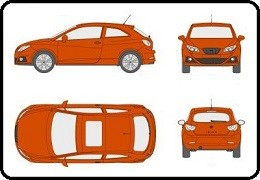This is an actual car. So, automobile engineers will work on their design, and based on the design, the product is manufactured and many cars are manufactured and sold in the market. Now, this design is a successful one.

Now the company is trying to make a new model of the same car. So, do you think they have to do the design work from the beginning or again from the scratch? No, they can carry out the same features in a new design.This is a new design. All the features have been borrowed from the previous design and extra features have been added. So, they can add multiple features they want to the old design. That means for making a new model which is matching with the first one, they don’t have to do the redesigning work. Just they can extend the existing design and add new features to that design to come up with a new design. This is an example of inheritance. Acquiring the features of an existing design into a new design is nothing but inheritance.

Now let us take another example. The electronics companies have a television model and they want to introduce a new model. So, they will not work out the new model from the scratch. Just they will extend the existing television model and add new features and launch a new model. Now, the same thing we want in software. In programming languages also, we want to do the same thing.

##### Inheritance in Programming Language:

Let us understand Inheritance in C++ Programming Language with an Example. Suppose we have a class called Rectangle having length and breadth as data members as follows.

class Rectangle{
int length;
};

Then we can create objects of class Rectangle as follows:

Rectangle r1, r2, r3;

Now, we want to write a class which is having the features same as Rectangle. But we don’t have to rewrite the code. For Example, we want to write a class for Cuboid. A cuboid is nothing but a rectangular shape but it is having one extra dimension that is height. So, we can write the Cuboid class as follows:

class Cuboid : Rectangle{
int height;
};

Here the Cuboid class is inheriting from the class Rectangle. We want everything that is inside Rectangle to be borrowed in class Cuboid also. Now objects of Cuboid can be created with various dimensions whatever we want. Let’s create objects of Cuboid class as follows

Cuboid c1, c2, c3;

Like other engineering, we have our design i.e. object of that design and then we have a new design i.e. the object of the new design and the new design is borrowing all the features of the existing design.

So, in this way, we can achieve reusability in programming which is the reusability of a design. We don’t have to redesign again from the beginning or scratch. So, we just need to inherit a new class from an existing class. This is the concept of inheritance which is the procedure of borrowing the features of an existing class into a new class. Here everything from the class Rectangle will be taken in class Cuboid. And then we added new features in the Cuboid.

##### Simple Example to Understand Inheritance in C++:

Now let us see a simple example of inheritance in C++. Please have a look at the following class.

class Base{
public:
int x;
void Show(){
cout << x;
}
};

Here we have written a class called Base. Inside this class, we have an integer type variable called x. Then we have a void type function called Show that will print the value of x in the console window. We have written everything as public here inside the Base class. Now we are writing another class called Derived as follows.

class Derived : public Base{
public:
int y;
void Display(){
cout << x << ” ” << y;
}
};

Here we have written another class called Derived. This Derived class is inherited from the Base class. The colon (:) operator is used when we are inheriting from a class. This colon (:) operator is used for showing the extension of a class. This is the method or syntax in C++ for inheriting from a class.

Then inside the class Derived, we have created an integer type variable called y. Next, we have created one void type Display function. Inside the Display function, we are printing the values of x and y. Here, we can access x as we are inheriting this Derived class from the Base class. Diagrammatically we can represent this as follows: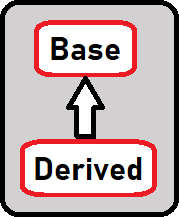Here, we have shown an upward arrow to show that the Derived class is inheriting from the Base class. Now let us create the objects of these classes in the main function and see what happens.

int main(){
Base b;
b.x = 20;
b.Show();
Derived d;
d.x = 3;
d.y = 5;
d.Show();
d.Display();
}

Here we have written some statements inside the main function. Let us understand them one by one. First, we have created an object of the Base class i.e. b. Then we have assigned the value of x to 20 using the object b. Then we have called the function Show which will print the value of x i.e. 20.

Next, we have created an object of the Derived class i.e. d. Then we have assigned the value of d’s x and d’s y as 3 and 5. Then we have called the Show and Display methods using the derived class d object. The Show function will print the value of x only and the Display function will print the values of x as well as y.

Here we have accessed the x and Show function through Derived class object d. This is because we have inherited the Derived class from the Base class. So, we can access the Base class data members and member function through the object of the Derived class. For a better understanding, please have a look at the below image.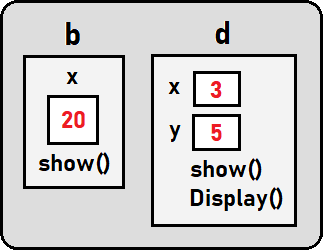This is a simple example showing how we can get the features of the existing class to a new class. This is called Inheritance in C++. Here, we are using two terms i.e. Base class and Derived class and the meaning of these two classes are as follows:

1. Sub Class/ Derived Class/ Child Class: The class that inherits properties from another class is called Subclass or Derived Class.
2. Super Class/ Base Class/ Parent Class: The class whose properties are inherited by a subclass is called Base Class or Superclass.

Now let us write the complete program in C++.

##### Example to Understand Inheritance in C++:
```#include <iostream>
using namespace std;

class Base
{
public:
int x;
void Show()
{
cout << x << endl;
}
};

class Derived : public Base
{
public:
int y;
void Display ()
{
cout << x << " " << y << endl;
}
};

int main()
{
Base b;
b.x = 20;
b.Show();

Derived d;
d.x = 3;
d.y = 5;
d.Show();
d.Display();
}
```
###### Output: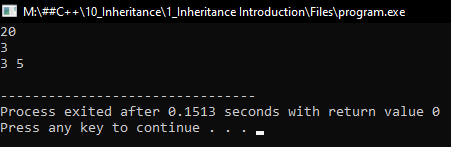##### What is Inheritance in C++?

Inheritance in C++ is a process in which one object acquires all the properties and behaviors of its parent object automatically. In this way, we can reuse, extend or modify the attributes and behaviors which are defined in other classes. In C++, the class which inherits the members of another class is called the derived class and the class whose members are inherited is called the base class.

##### Advantages of Inheritance in C++:

Code reusability: We can reuse the members of the parent class or base class in the child class or derived class. So, there is no need to re-define the members again in the child class. So less code is required in the class.

##### Types Of Inheritance in C++:

C++ supports five types of inheritances. They are as follows:

1. Single Inheritance
2. Multiple Inheritances
3. Hierarchical Inheritance
4. Multilevel Inheritance
5. Hybrid Inheritance
##### Syntax to use Inheritance in C++:

A Derived class in C++ is defined as the class derived from the base class. The Syntax of the Derived class in C++ is given below:

class Derived_Class_Name : Access-Mode Base_Class_Name
{
//Body of the Derived Class.
}

Here,

1. Derived_Class_Name: It is the name of the Derived Class.
2. Access-Mode: It specifies whether the features of the base class are publicly inherited or privately inherited. It can be public or private.
3. Base_Class_Name: It is the name of the Base class.
##### Real-time Example to Understand Inheritance in C++:

Now let us look at one real-time example to understand inheritance in C++. Please have a look at the following Rectangle class.

```class Rectangle
{
private:
int length;
public:
Rectangle (int r = 0, int b = 0);
int getLength ();
void setLength (int l);
int area ();
int perimeter ();
};```

Here we have a class called Rectangle. The Rectangle will have dimensions that are length and breadth as shown in the below image.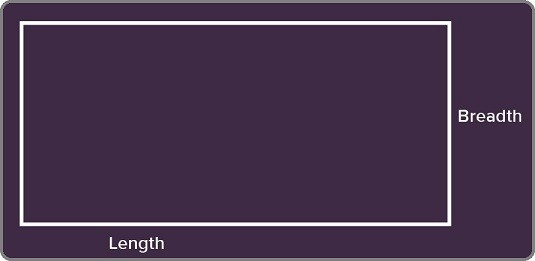To the same rectangle if we add height as a dimension then it becomes a Cuboid.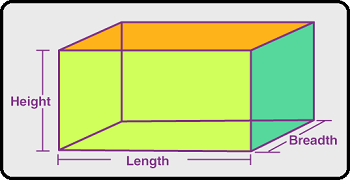So, Cuboid has an extra dimension that is height. We have a class called Rectangle in which we have all the required member functions. For simplicity, we have written only the signature. At the time of coding, we will give the implementations.

Now we want a class for Cuboid. So let us write a class Cuboid inheriting from the Rectangle class so that it will get the length and breadth plus it can have its own height data members. Let us see how we write this class Cuboid. Please have a look at the below code.

```class Cuboid:public Rectangle
{
private:
int height;
public:
Cuboid (int l = 0, int b = 0, int h = 0)
{
height = h;
setLength (l);
}
int getHeight ();
void setHeight (int h);
int volume ()
{
return getLength () * getBreadth * height;
}
};```

Here we have written the class Cuboid. This class is publically inheriting from class Rectangle. Later we will discuss what are public, private, and protected access specifiers in inheritance.

Then we have created an integer type variable that is height in the private section. We just created height because we are inheriting this class from Rectangle. That means it has already the length and breadth.

In the public section, we have created parameterized constructor for the Cuboid class. This will also take default arguments. If the user doesn’t pass anything in the constructor, then the constructor will take default values i.e. 0. So, this constructor will also act as the default constructor.

Inside the constructor, we have assigned the values of length, breadth, and height. First, we have assigned the value of height to h. Next, we cannot directly assign values for length and breadth like length = l or breadth= b. We cannot do this as these members are private in the Rectangle class so these private members are not accessible directly. So, what can we do? We can assign them by using the setLength and setBreadth member functions. We have just passed the l and b as parameters to setLength and setBreadth functions.

Next, we have declared getHeight function of return type int. And setHeight function of return type void. We have just declared these functions not implemented them. Then we have the Volume function which will return the calculated volume of Cuboid. Inside this method, we have used getLenght and getBreadth function to get the value of length and breadth. As we cannot directly access these variables so we have used those functions.

Now let us write the main method in which we will create an object of class Cuboid class as follows.

int main(){
Cuboid c(10, 5, 3);
cout << c.getLength();
cout << c.volume();
}

Inside the main function, we have created an object C of class Cuboid. We have set length, breadth, and height as 10, 5, and 3. Then we printed the value of C’s length. And then printed the cuboid volume. Now let us write the complete program.

Complete Example Code to Understand Inheritance in C#:

```#include <iostream>
using namespace std;

class Rectangle
{
private:
int length;
public:
Rectangle();
Rectangle(int l, int b);
Rectangle(Rectangle & r);
int getLength()
{
return length;
}
{
}
void setLength(int l);
int Area();
int Perimeter();
};

class Cuboid : public Rectangle
{
private:
int height;
public:
Cuboid(int h)
{
height = h;
}
int getHeight()
{
return height;
}
void setHeight(int h)
{
height = h;
}
int Volume()
{
return getLength() * getBreadth() * height;
}
};

Rectangle::Rectangle()
{
length = 1;
}

Rectangle::Rectangle(int l, int b)
{
length = l;
}

Rectangle::Rectangle(Rectangle & r)
{
length = r.length;
}

void Rectangle::setLength(int l)
{
length = l;
}

{
}

int Rectangle::Area()
{
}

int Rectangle::Perimeter()
{
return 2 * (length + breadth);
}

int main()
{
Cuboid c(8);
c.setLength(2);
cout << "Volume is " << c.Volume() << endl;
cout << "Area is " << c.Area() << endl;
cout << "Perimeter is " << c.Perimeter() << endl;
}
```
###### Output: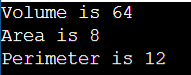1.